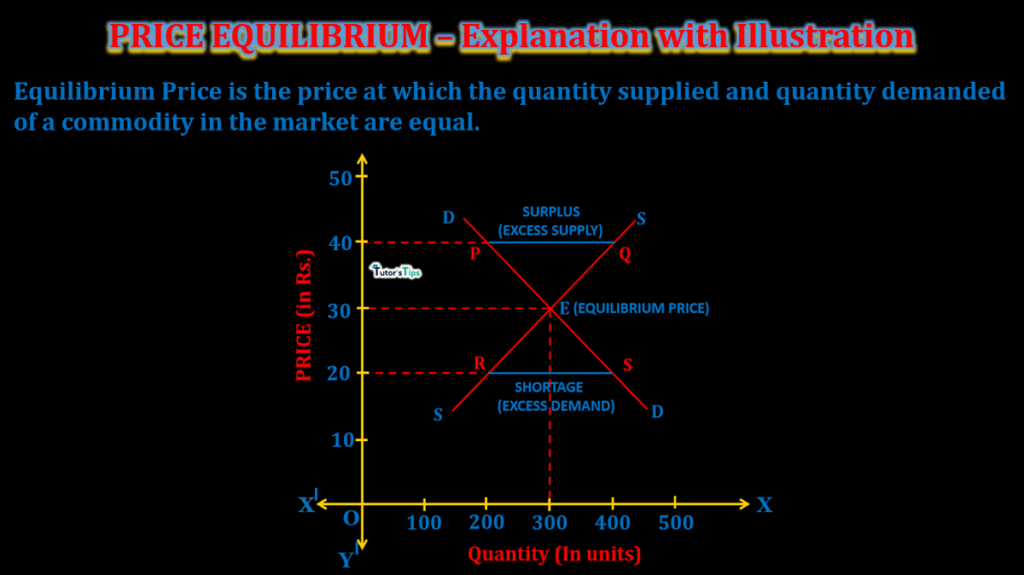# Price Equilibrium – Explanation with IllustrationPrice equilibrium is the price where the quantity supplied and demanded of a commodity in the market are equal.

## What is Price Equilibrium?

The price at which buyers are ready to buy and sellers are ready to sell the goods and services in the market is known as Equilibrium Price. The point where demand and supply are equal is the price equilibrium point.

PRICE EQUILIBRIUM :

where Demand of a commodity  =  Supply of a commodity

Demand refers to the willingness and ability of buyers to purchase a commodity in the market. It is indicated by the demand schedule or demand curve.

Supply refers to the availability of a commodity by all firms in the market and indicated by supply schedule and supply curve.

The oversupply of commodities results in a decrease in price, causing a rise in demand. This effect of balancing the demand and supply in the market is shown by price equilibrium.

In other words, the point where the demand and supply curve intersect each other is known as the price equilibrium point. There is no excess demand or excess supply in the market.

## Explanation with Illustration :

We can understand this with the following illustration:

Suppose, the demand and supply schedule for a commodity ‘X’ at different prices in the market is as follows:

 Price of commodity ‘X'(In Units) Quantity demanded of commodity ‘X'(In Units) Quantity Supplied of Commodity ‘X'(In Units) 50 100 500 40 200 400 30 300 300 20 400 200 10 500 100

The above table shows that at price Rs.50, the quantity demanded is 100 units whereas quantity supplied of commodity ‘X’ is 500 units. At this price, there is excess supply as the sellers have excess stock to offer. It means that sellers are willing to sell more than what consumers want to buy. This excessive supply forces the price to lower down in the market. Suppose the price falls to Rs 40, it leads to a rise in quantity demanded to 200 units and fall in supply to 400 units. Even at this price, the supply of commodity ‘X’ > its demand, thus the situation of excess supply prevails in the market. It again forces the price to fall, resulting in a new price at Rs 30. This fall in price leads to an increase in demand to 300 units and a decrease in supply to 300 units. Thus, Rs.30 is the equilibrium price as the quantity demanded and supplied are equal i.e.300 units.

Similarly, at any price below the equilibrium price, there would be excess demand. It means that there is a shortage of stock in the market as the quantity demanded by buyers is more than what sellers want to sell. At the equilibrium price, there is no excess demand and supply.

### Graphical Representation :

In fig, X-axis shows the quantity demanded by buyers and quantity supplied by sellers of commodity ‘X’ whereas Y-axis shows the different price levels. DD is the demand curve and SS is the supply curve. The point E is the equilibrium point where both curves intersect each other which implies Demand= Supply. This point shows that the equilibrium price is Rs.30 and the equilibrium quantity is 300 units. If the price is more than equilibrium price i.e. Rs.40, it results in excess supply and shown by points P & Q where Supply is 400 units whereas Demand is only 200 units. The excess supply pressurizes the price to fall down in the market which leads to an increase in demand (movement along the curve from P to E)   and a decrease in supply (movement along the supply curve from Q to E). At point E, the excess supply is eliminated and results in demand = supply=300 units.

Likewise, if the price is less than the equilibrium price i.e. Rs 20, it results in excess demand and shown by points R & S where Supply is 200 units and Demand is 400 units. The excess demand forces the price to rise in the market which leads to an increase in supply(movement along the supply curve from R to E)  and a decrease in demand (movement along the supply curve from S to E). At point E, the excess demand is eliminated and results in demand = supply=300 units. Thus, the market is cleared and the equilibrium price is prevailing.

Thanks!!!

Comment if you have any question.

Check out Business Economics Books@ Amazon.in

This site uses Akismet to reduce spam. Learn how your comment data is processed.